# Review — Improved Techniques for Training GANs

## Improved DCGAN, Invented Inception Score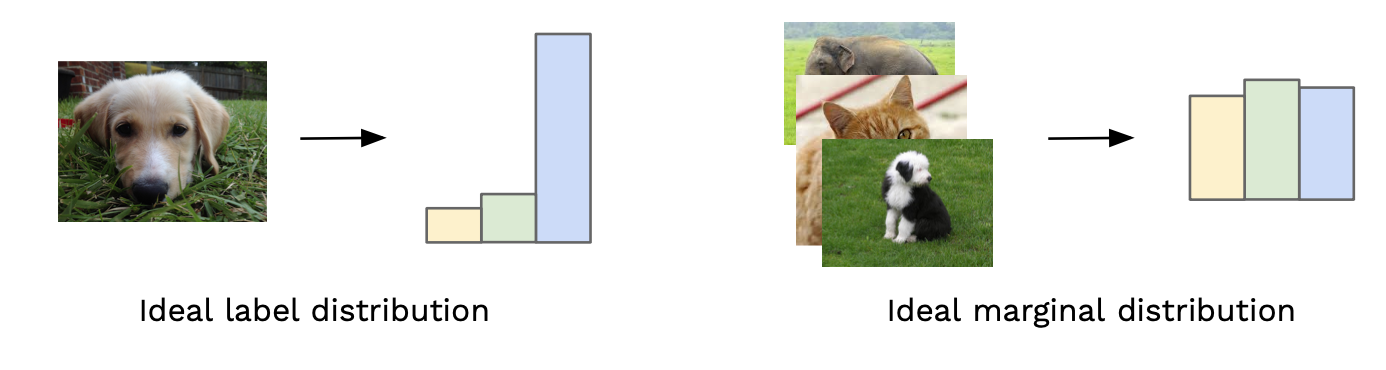Left: Low Inception Score, Right: High Inception Score (Image from David Mack’s Medium Story)

Improved Techniques for Training GANs
Improved
DCGAN, Inception Score, by OpenAI
2016 NIPS, Over 6000 Citations (Sik-Ho Tsang @ Medium)
Generative Adversarial Network, GAN, Inception Score, Semi-Supervised Learning, Image Classification

• A variety of new architectural features and training procedures is proposed, two applications of GANs are focused: semi-supervised learning, and the generation of images that humans find visually realistic.
• One of the major inventions in this paper is the Inception score. This score is to evaluate the quality of generative models for images, which is used by many papers.
• And this is the first paper to generate large-size (128×128) ImageNet images.

# Outline

1. Toward Convergent GAN Training
2. Assessment of Image Quality and Inception Score (IS)
3. Semi-Supervised Learning
4. Experimental Results

# 1. Toward Convergent GAN Training

• Training GANs is difficult. A modification to discriminator parameters θ(D) that reduces the discriminator cost J(D) can increase generator cost J(G), and a modification to generator parameters θ(G) that reduces J(G) can increase J(D). Gradient descent thus fails to converge for many games.
• For example, when one player minimizes xy with respect to x and another player minimizes -xy with respect to y, gradient descent enters a stable orbit, rather than converging to x=y=0.

## 1.1. Feature Matching

• Feature matching addresses the instability of GANs by specifying a new objective for the generator that prevents it from overtraining on the current discriminator.
• Letting f(x) denote activations on an intermediate layer of the discriminator, the new objective for the generator is defined as:
• while the discriminator, f(x), are trained in the usual way.

## 1.2. Minibatch Discrimination

• One of the main failure modes for GAN is for the generator to collapse to a parameter setting where it always emits the same point.
• Because the discriminator processes each example independently, there is no coordination between its gradients, and thus no mechanism to tell the outputs of the generator to become more dissimilar to each other. After collapse has occurred, the discriminator learns that this single point comes from the generator, but gradient descent is unable to separate the identical outputs.
• Let f(xi) denote a vector of features for input xi, produced by some intermediate layer in the discriminator.
• The vector f(xi) is then multiplied by a tensor T, which results in a matrix Mi as shown above.
• The L1-distance is then computed between the rows of the resulting matrix Mi across samples where a negative exponential is used.
• The output o(xi) for this minibatch layer for a sample xi is defined as the sum of the cb(xi, xj)’s to all other samples:
• The output o(xi) of the minibatch layer is concatenated with the intermediate features f(xi), and it is fed into the next layer of the discriminator.
• The discriminator is still required to output a single number for each example indicating how likely it is to come from the training data.

## 1.3. Historical Averaging

• Each player’s cost is modified to include a term:
• where θ[i] is the value of the parameters at past time i.

## 1.4. One-sided Label Smoothing

• Label smoothing is used, similar to Inception-v3.
• Replacing positive classification targets with α and negative targets with β, the optimal discriminator becomes:
• But smoothing only the positive labels to α, leaving negative labels set to 0.

## 1.5. Virtual Batch Normalization (VBN)

• Each example x is normalized based on the statistics collected on a reference batch of examples that are chosen once and fixed at the start of training, and on x itself. The reference batch is normalized using only its own statistics.
• VBN is computationally expensive, and thus only applying VBN on generator.
• (For these improving techniques, authors only described verbally or briefly, for the actual operation, it is needed to read the codes.)

# 2. Assessment of Image Quality and Inception Score (IS)

## 2.1. Human Annotator

• Amazon Mechanical Turk (MTurk), using the web interface, is used, in which annotators are asked to distinguish between generated data and real data.
• However, human annotation is expensive, especially for large dataset. As an alternative to human annotators, an automatic method to evaluate samples, is proposed, as described below.

## 2.2. Inception Score (IS)

• The Inception model, Inception-v3, is used for every generated image to get the conditional label distribution p(y|x).
• Images that contain meaningful objects should have a conditional label distribution p(y|x) with low entropy.
• Moreover, the model is expected to generate varied images, so the marginal ∫p(y|x=G(z))dz should have high entropy.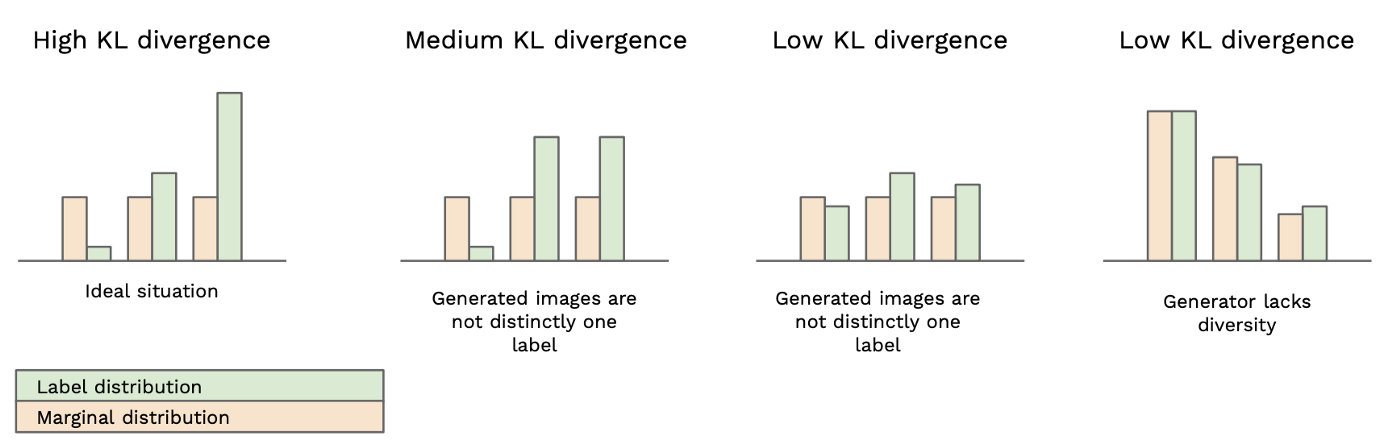KL Divergence Conditions (Image from David Mack’s Medium Story)
• Combining these two requirements, the metric that we propose is:
• where exponentiating is to make the values easier to compare.

it is found that this is a good metric for evaluation that correlates very well with human judgment. It’s important to evaluate the metric on a large enough number of samples (i.e. 50k) as part of this metric measures diversity.

# 3. Semi-Supervised Learning

• Consider a standard classifier for classifying a data point x into one of K possible classes. Such a model takes in x as input and outputs a K-dimensional vector.
• Semi-supervised learning with any standard classifier is performed by simply adding samples from the GAN generator G to the dataset, labeling them with a new “generated” class y=K+1, and correspondingly increasing the dimension of our classifier output from K to K+1, to supply the probability that x is fake.
• Assuming half of dataset consists of real data and half of it is generated (this is arbitrary), the loss function for training the classifier then becomes:
• In terms of G and D, Lunsupervised becomes:

# 4. Experimental Results

## 4.1. MNIST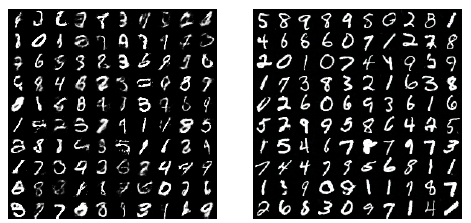Samples generated during semi-supervised training on MNIST with feature matching (Left) and minibatch discrimination (Right)
• D & G: Networks have 5 hidden layers each. Weight normalization and Gaussian noise are added to the output of each layer of the discriminator.
• Left: samples generated by model with feature matching during semi-supervised training. Samples can be clearly distinguished from images coming from MNIST dataset.
• Right: Samples generated with minibatch discrimination. Samples are completely indistinguishable from dataset images.
• However, semi-supervised learning with minibatch discrimination does not produce as good a classifier as does feature matching.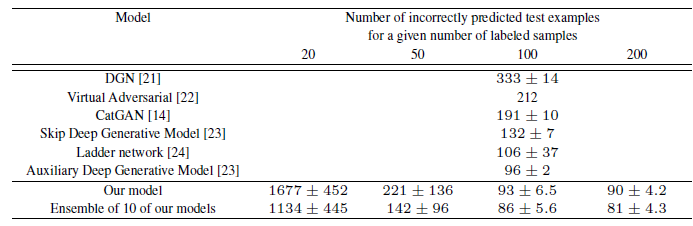Number of incorrectly classified test examples for the semi-supervised setting on permutation-invariant MNIST
• Considering setups with 20, 50, 100, and 200 labeled examples, the proposed GAN obtains the best results on 100-labeled-example case.

## 4.2. CIFAR-10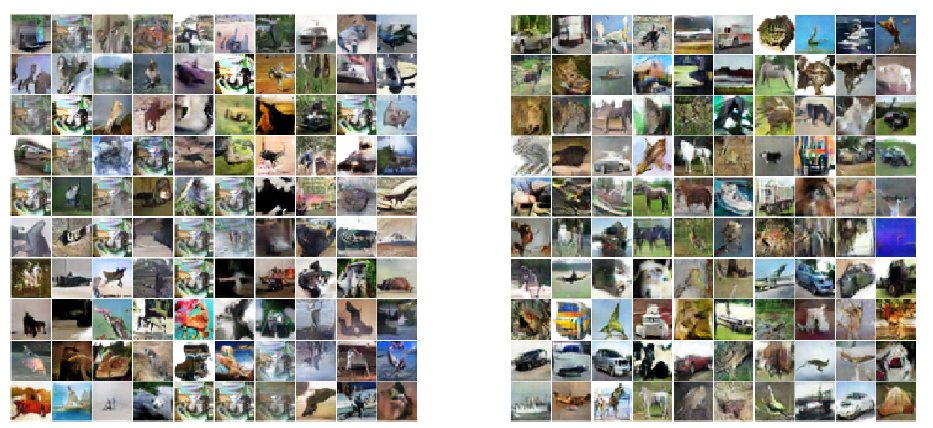Samples generated during semi-supervised training on CIFAR-10 with feature matching (Left) and minibatch discrimination (Right)

Again, the proposed model obtains the best results outperforms such as Ladder Network.

## 4.3. SVHN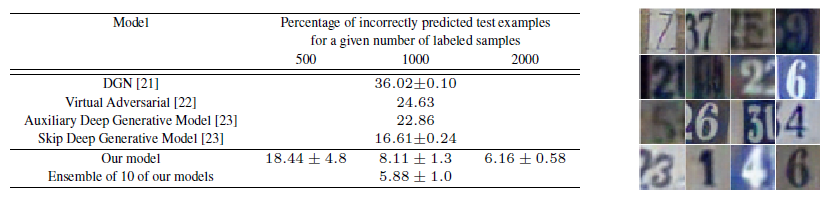(Left) Error rate on SVHN. (Right) Samples from the generator for SVHN
• The same architecture and experimental setup are used as for CIFAR-10.
• Similar trends are obtained, outperforms such as VAT .

## 4.4. Inception Score (IS)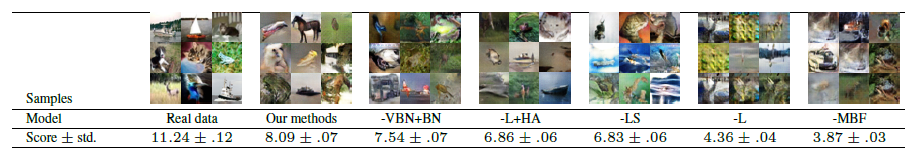Table of Inception scores for samples generated by various models for 50; 000 images.
• The Inception score correlates well with the subjective judgment of image quality.
• Samples from the dataset achieve the highest value.
• All the models that even partially collapse have relatively low scores.
• “Our methods” includes all the techniques described in this work, except for feature matching and historical averaging.

The Inception score should be used as a rough guide to evaluate models that were trained via some independent criterion.

## 4.5. ImageNet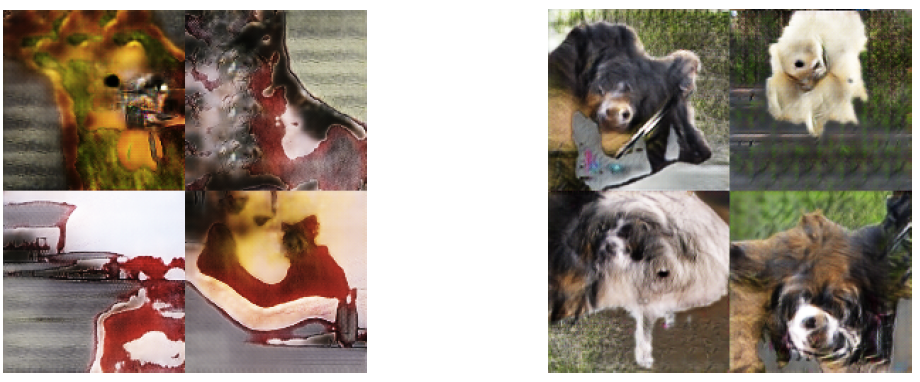Samples generated from the ImageNet dataset. (Left) Samples generated by a DCGAN. (Right) Samples generated using the techniques proposed in this work.
• To the author’s knowledge, no previous publication has applied a generative model to a dataset with both this large of a resolution (128×128) and this large a number of object classes.

The proposed Inception Score (IS) has been used in many papers.

## References

[2016 NIPS] [Improved DCGAN, Inception Score]
Improved Techniques for Training GANs

[Medium Story from David Mack] [Inception Score]
A simple explanation of the Inception Score

## Generative Adversarial Network (GAN)

Image Synthesis: 2014 [GAN] [CGAN] 2015 [LAPGAN] 2016 [AAE] [DCGAN] [CoGAN] [VAE-GAN] [InfoGAN] [Improved DCGAN, Inception Score] 2017 [SimGAN] [BiGAN] [ALI] [LSGAN] [EBGAN] 2019 [SAGAN]

## Semi-Supervised Learning

2004-2016 … [Improved DCGAN, Inception Score] … 2020 [BiT] [Noisy Student] [SimCLRv2] [UDA] [ReMixMatch] [FixMatch] 2021 [Curriculum Labeling (CL)] [Su CVPR’21]

--

--